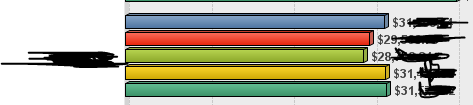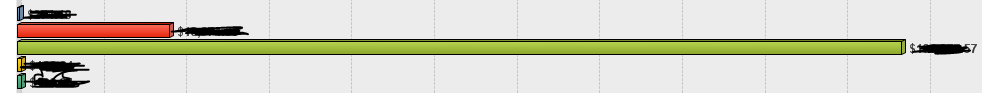# New to QlikView

Discussion board where members can get started with QlikView.

New Contributor

## Display Graph if Spike is noticed

Hi,

I have built a bar graph which gives me the Usage per country per month.

Most of the times, the usage is average for each month, but if for some reason there is a spike in usage for a country for a particular month compared to other months, only then the graph should display those countries. How can i achieve this?

Ex:

In the below graph that i have, data is displayed for last 4 months and the values are average for one country. As its average usage for all the months, this country should not be displayed.But for another country, there was a major spike in one month, so this should be displayed in the Bar.How can i achieve this?

I am looking only for those countries which have sudden peaks in usage compared to previous months. Something like if the usage is greater than 30% of the average for a particular month, only then display the graph.

Thanks

Taher

4 RepliesPartner

## Re: Display Graph if Spike is noticed

Hi,

Try Below,

=if(avg({<Year={\$(=Max(Year))}>} Usage) >avg({<Year={\$(=Max(Year)-1)}>} Usage),avg(Usage))MVP

## Re: Display Graph if Spike is noticed

May be try this?

If(Sum(usage)>(Sum({<Year={\$(=Max(Year))}>} usage) * 0.3), Sum(usage))

Before develop something, think If placed (The Right information | To the right people | At the Right time | In the Right place | With the Right context)
New Contributor

## Re: Display Graph if Spike is noticed

Hi Anil,

I copied the below under expressions in the graph, but didn't notice any difference:

If(Sum(RES_AMOUNT)/1000000>(Sum({<MONTH_YEAR={\$(=Max(MONTH_YEAR))}>} RES_AMOUNT/1000000) * 0.3), Sum(RES_AMOUNT)/1000000)

I am suspecting the 'MONTH_YEAR' which holds values as:

APR-2018

MAY-2018

is not working. Any idea what could be wrong?

New Contributor

## Re: Display Graph if Spike is noticed

Hi Aruna

Hi Anil,

I copied the below under expressions in the graph, but didn't notice any difference:

=if(avg({<MONTH_YEAR={\$(=Max(MONTH_YEAR))}>} RES_AMOUNT/1000000) >avg({<MONTH_YEAR={\$(=Max(MONTH_YEAR)-1)}>} RES_AMOUNT/1000000),avg(RES_AMOUNT/1000000))

I am suspecting the 'MONTH_YEAR' which holds values as:

APR-2018

MAY-2018

is not working. Any idea what could be wrong?